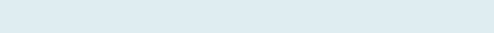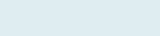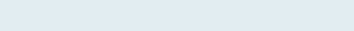In this article, you will understand adding numbers with a criterion by following six examples from simple to complex. For resolving our queries, we will use Excel SUMIF and SUMPRODUCT functions.

Excel categorizes SUMIF and SUMPRODUCT function under Math functions. This function adds all numbers in a range of cells that meet specific criteria.

In this post, you will go through Six distinct examples for a stronger understanding of adding value in different scenarios.

The key example which will you cover here are:

## Sumproduct with More than one Headings

Let’s first refresh our understanding of the SUMIF function.The SUMIF formula in Excel takes three arguments out of which one is optional.

Remember: Excel starts calculating innermost parenthesis than work outward. You can also debug the formula using a shortcut F9 function key or under the formulas, tab click on Evaluate formula.## Sum One Column based on Another

Adding numbers referencing to the next column where some cells are empty and remaining fills with text, number, dates, etc. In our case we have numbers.

Query:

In this example, we have the columns Receipt number, Sales and Payment left. Our task is to add all Sales (column C) if the value in the range (D4 to D13) and ignore if empty.

Formula to implement:

=SUMIF(D4:D13,”<>”,C4:C13)

Result =43351

Here, we have selected range from D4 to D13, where criteria are “<>” (not equal) and then sum range is C4 to C13 if TRUE, therefore it adds all given range which is not empty.

If you want to reverse the condition and want to add values based on the empty cells in next column the criteria parameter will change from “<>“ to “” (empty). The new formula will sum all sales numbers in a column (C) with reference to the column (D) with no number or ignoring any text in column (D) range E4 to E13. Check the below formula,

=SUMIF(D4:D13,””,C4:C13)

Return: 41110   Added cells only if the next cell is empty.

When you want to calculate the column’s SUM that is filled with numbers if some cells contain no numbers (text) then display text. We can implement a function to check errors along with the SUMIF formula.

Query:

In this example, we have columns Item code, and Units. Our task is to sum similar code units’ (Cumulative) values if there is text and no numbers then display text “BULK”.

Formula to implement:

=IFERROR(1/(1/SUMIF(\$E\$3:\$E\$17,E3,\$F\$3:F\$17)),”BULK”)

It will return cumulative numbers (successive additions) of the same Item code other than will show the text as a result if ‘Bulk’. Like here in Cell G3 it displays text “BULK” and Cell G4 it displays 15 as there is only one code, whereas in cell G5 & G10 it displays 43 (23+20) as there are two similar codes (TU294).

## Average only if similar Text Heading

When you want to know the average of a column with similar text in multiple rows. Here we will implement the COUNTIF formula along with SUMIF to know the average.

Query:

In this example, we have columns Product and Quantity (option 1, option 2, option 3). Our task is to add all numbers of the product (Watch) within option 1 category and then divide it by counting how many times Watch occur (3), to know the average quantity of mobile in the ‘option 1’ category.

Formula to implement:

=SUMIF(\$C\$4:\$C\$13,I\$6,\$D\$4:\$D\$13)/COUNTIF(\$C\$4:\$C\$13,I\$6)

Result: 9.67

(14+11+4)= 29, count =3,

(29/3)= 9.67

It first adds the number based on the criteria “Watch” in the given range C4 to C13 then it divides after counting on the basis of the condition (Watch) in the given range. As mobile comes three times in range C4 to C13 so it divides by 3 after adding all watch in option 1 of quantity.

Decrease the decimal places under the Excel HOME tab.

## Sum Specific Column with similar Text

Adding respective column values with similar text in multiple rows in Excel. We will use INDEX and MATCH along with the SUMIF formula.

QUERY:

In this example, we have columns Product and Quantity of option 1, option 2, option 3. Our task is to know the lookup value of Mobile in Option 3 range, and then return the addition of specific (TRUE) cell given by “Mobile” of column (C) product.

Formula to implement:

=SUMIF(\$C\$4:\$C\$13,I\$6,INDEX(\$D\$4:\$F\$13,,MATCH(F\$3,\$D\$3:\$F\$3,)))

Result: 46(18+12+16)= 46      ‘Only If criteria is TRUE

MATCH function first returns the position of column “option 3” in the given range D3 to F3 i.e. ‘3′ and INDEX returns all value of Option 3.  Both Excel functions together make the selection of Options dynamic.

NoteCreate a Drop-down list for easy working with our formula in such a scenario.

## Sumproduct with two Ranges and Items

Adding a column if similar text then returns the sum of the product. For this, we will apply SUMPRODUCT along with the SUMIF formula.

QUERY: We have price and invoice quantity. Our task is to add the quantity of the given product and then multiply them with price in another table, returning the total invoice value.

Formula to implement:

=SUMPRODUCT(SUMIF(B7:B10,B3:B4,C7:C10),C3:C4)

Result: 430

(17+3)= 20, (2+1)= 3           ‘Add Pen and Pencil Quantity(20*20)= 400, (10*3)= 30    ‘Multiply Price and Quantity

(400+30)= 430

Here first we add all the numbers on the basis of the given criteria i.e. “pen and pencil” in the given sum_ range B7 to B10 and then multiply the added number with the price (column C) and gives the sum of products.

## Sumproduct with More than one Headings

When you want to add multiple values in columns and rows if it is with the same name, apply the below formula. We used here SUMPRODUCT formula.

Query:

We have columns Name, line 1, line 2, line 3, line 4, line 1, line 3, line 6, and line 7. Our task is to sum all the numbers with similar Name (Line 1) and returns the sum of the product. Here we have duplicate rows and columns name, so we have 2 criteria, one for rows and one for columns. In such a case, we will be using Excel’s SUMPRODUCT function.

Formula to implement:

=SUMPRODUCT((\$F\$3:\$F\$11=G\$15)*(\$G\$2:\$N\$2=H\$14),\$G\$3:\$N\$11)

Result: 282

It returns TRUE if “Joe” or “Line 1” in the given range otherwise return FALSE.(63+50+69+13+74+13)= 282          ‘Add only TRUE with Joe

First, it checks from the given range F3 to F11 where the criteria name (G15) “Joe” so it returns TRUE otherwise FALSE if the name is not Joe. Then it checks the second criteria “line 1” it also returns TRUE that meets criteria in the range G2:N2 otherwise it returns FALSE. After that, it adds all numbers in the range G3 to N11 which meets both the criteria Joe and line 1 comparing with columns and rows.

You can also give a name to each sheet or range for better understanding and easy to recognize by clicking the formulas tab and then click define name range for naming the set of cells.

Note: &” operator in Excel is a type of concatenate which is used to join two strings, values or results.